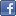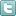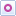EE Times-India > Memory/Storage

Memory/Storage# Grasp floating-point data in embedded software

Posted: 05 Oct 2015Print Version

Keywords:floating point  integer arithmetic  CPUs  coding  C

Although many embedded applications can be implemented using integer arithmetic, there are times when the ability to deal with floating point (real) numbers is required. This article looks at the details of floating point operations, when floating point should and should not be used, some of the pitfalls of its use and how its use may sometimes be avoided.

Floating point and integers
Nowadays, most embedded systems are built using 32bit CPUs. These devices give plenty of scope for performing the arithmetical processing required for various applications. Calculations can be performed on signed or unsigned integers and 32 bits gives a good range of values: ± 2 billion or up to 4 billion respectively. Extending to 64 bits is reasonably straightforward.

If you need to stray outside of these ranges of values or perform more sophisticated operations, then you need to think in terms of floating point and this presents a selection of new challenges.

The concept of a floating point number is simple enough—the value is stored as two integers: the mantissa and the exponent. The number represented is the mantissa multiplied by 2 to the power of the exponent. Typically, these two integers are stored in bit fields in a 32bit word, but higher precision variants are also available. The most common format is IEEE 754-1985.

The clear benefit of using floating point is the wide range of values that may be represented, but this comes at a cost of extra care when coding and some trade-offs:

Performance. Floating point operations take a lot of time compared with integers. If the processing is done in software, the execution time can be very long indeed. Hardware floating point units speed up operations to a reasonable extent.

Precision. Because of the way that values are represented in floating point, a value may not be exactly what you expect. For example, you may anticipate a variable having the value 5.0, but it actually is 4.999999 This need not be a problem, but care is needed in coding with floating point.

Coding with floating point
Because of the intrinsic lack of absolute precision in floating point operations, code like this would clearly be foolish:

if (x == 3.0)
...

as x may never be precisely 3.0.

Similarly, coding a loop like this might produce unexpected results:

for (x=0.0; x<5.0; x++)
...

You would expect the loop to be performed 5 times for x values 0.0, 1.0. 2.0, 3.0 and 4.0 This might work, but it is quite possible that an extra iteration will occur for x being 4.999999.

The solution is to use an integer loop counter:

for (i=0,x=0.0; i<5; i++,x++)
...

Binary floating point
Most embedded developers understand the binary representation of numbers – even if many are less than 100% comfortable with its use on a daily basis. Binary integers are easy enough: the digits, going from right to left, represent 20, 21, 22, 23 and so on. So, the number 10011 is 1 + 2 + 16 = 19.

It is less common to see floating point numbers represented in binary, but just as useful to understand how they work. The left of the binary point (not the decimal point now!) – the whole part of the number – is represented like a binary integer. That is obvious. The more confusing part is to the right of the binary point – the fractional part of the number. Here the digits represent 2-1, 2-2, 2-3, 2-4 (1/2, 1/4, 1/8, 1/16 ...) and so on. So, the number 0.11011 is 0.5 + 0.25 + 0.0625 + 0.03125 = 0.84375

If you want to play with such numbers, here is a simple C function to display a float (which is less than 1) in binary:IEEE 754-1985 format
There are countless ways that floating point numbers might be represented in a computer. For example, the binary point could be located at an arbitrary point in a 32bit word and the binary pattern of digits interpreted accordingly. So, if the bottom 8 bits were to be designated the fraction, the value 0x00000280 would represent 2.5 (in decimal). Here it is in binary (I included the binary point):

0000 0000 0000 0000 0000 0010 . 1000 0000

 Related Articles Editor's Choice
Comment on "Grasp floating-point data in embedde..."

Top Ranked Articles

WebinarsVisit Asia Webinars to learn about the latest in technology and get practical design tips.

Search EE Times India
Services

Go to topConnect on FacebookFollow us on TwitterFollow us on Orkut
﻿

Back to Top